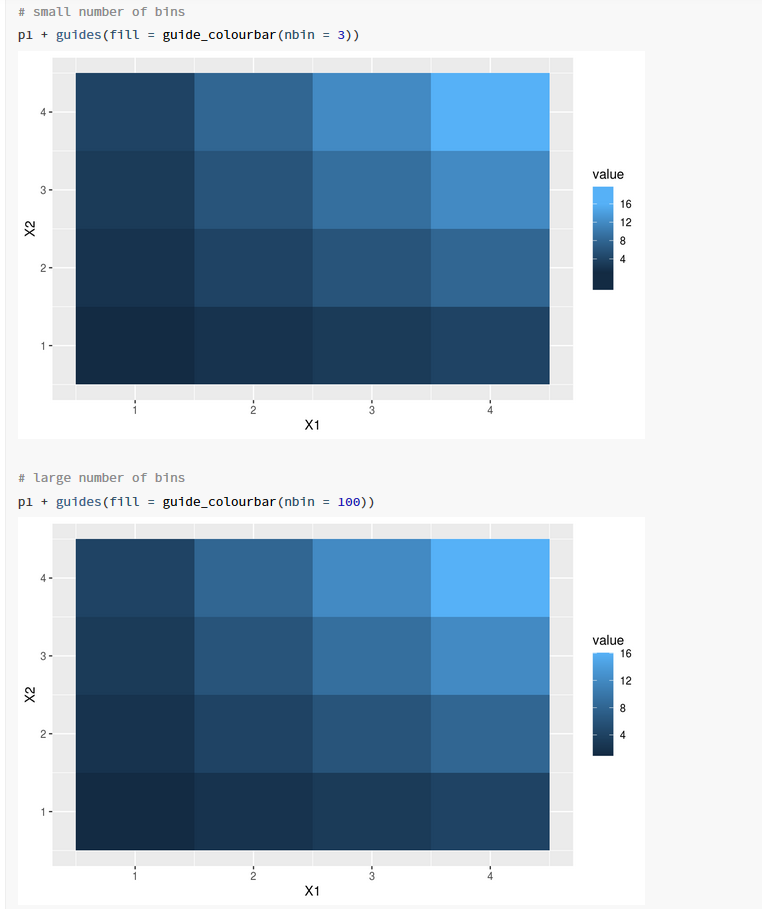{{ message }}
/ ggplot2 Public

# Feature request: enable expand arguments for continuous color and fill scales.#3508

Closed
opened this issue Aug 29, 2019 · 9 comments · Fixed by #3521
Closed

# Feature request: enable expand arguments for continuous color and fill scales. #3508

opened this issue Aug 29, 2019 · 9 comments · Fixed by #3521

### atusy commented Aug 29, 2019

Unlike scales for continuous x or y (e.g., `scale_x_continuous`), those for color or fill (e.g., `scale_color_gradient`) is not supporting `expand` option.
At least, I want `expand = c(0, 0)` to work so that color bars exactly represents the range of color or fill mappings.

The example below shows that range of color bar is wider than the range specified in `limits` argument even if `expand = c(0, 0)` is specified.

@yutannihilation figured out this is caused by

Line 336 in 047b528

 tick_pos <- rescale(guide\$key\$.value, c(0.5, guide\$nbin - 0.5), guide\$bar\$value[c(1, nrow(guide\$bar))]) * barlength / guide\$nbin

```x <- c(0, 1)
ggplot2::qplot(x, x, color = x) +
ggplot2::scale_color_gradient(breaks = x, limits = x, expand = c(0, 0)) +
ggplot2::guides( # Just for the visibility
color = ggplot2::guide_colorbar(
barheight = grid::unit(1, "npc") - grid::unit(4, "line"),
ticks.linewidth = 2
)
)```Created on 2019-08-29 by the reprex package (v0.3.0)

### yutannihilation commented Aug 30, 2019

 @yutannihilation figured out this is caused by (I talked with atusy outside of GitHub) Sorry, probably my explanation was not exact; you don't need `expand` argument because the colour scale is not expanded. The ticks are shifted by `0.5` because these are the places they should be as the gradient by `grid::rasterGrob()` is interpolated between the middles of the tiles, not between the edges of the tiles. For example, you can see the gradation happens only between the center of the black and the center of the pink: ```library(grid) g <- matrix(hcl(0, 100, c(0, 100)), nrow=2, ncol=1) # interpolated grid.newpage() grid.raster(g, x = 0.25, width = 0.5, height = 1) grid.raster(g, x = 0.75, width = 0.5, height = 1, interpolate = FALSE)```Created on 2019-08-30 by the reprex package (v0.3.0) So, the short answer would be "you don't need `expand`, the colorbar shows the exact range of the values," but I agree this might be a bit confusing to see the bar runs over the ticks... @thomasp85 @clauswilke Do you know there's some tricks to chop a `rasterGrob` at the very start and end of the gradient? Can we use `clipGrob`?

### clauswilke commented Aug 30, 2019

 I was confused by this bug report because I'm certain the color scale is not expanded. The trick to fix this issue is to increase the value of `nbin`, e.g. to 200. The default is too low. I've thought about fixing this properly but the math for axis placement gets quite involved and increasing `nbin` is just so much easier. ```library(ggplot2) x <- c(0, 1) # default nbin = 20, looks like the color scale is expanded ggplot(data = data.frame(x = x), aes(x, x, color = x)) + geom_point() + scale_color_gradient( breaks = x, limits = x, guide = guide_colorbar( barheight = grid::unit(1, "npc") - grid::unit(4, "line"), ticks.linewidth = 2 ) )``````# with nbin = 200, the effect disappears ggplot(data = data.frame(x = x), aes(x, x, color = x)) + geom_point() + scale_color_gradient( breaks = x, limits = x, guide = guide_colorbar( barheight = grid::unit(1, "npc") - grid::unit(4, "line"), ticks.linewidth = 2, nbin = 200 ) )```Created on 2019-08-29 by the reprex package (v0.3.0)

### yutannihilation commented Aug 30, 2019

 Ah, thanks! Since this is fundamentally a matter of resolution, `nbin` solution makes sense to me. The default is too low. Agreed. Do you think we can increase the default? It will break visual tests here and there, but I feel it's worth breaking.

### clauswilke commented Aug 30, 2019

 I think that's a question for @hadley.

### hadley commented Aug 30, 2019

 It's fine with me.

### yutannihilation commented Sep 1, 2019

 Thanks, then I'll create a PR for this. (Yet to investigate how many `nbin` is the best, though...)

### yutannihilation commented Sep 7, 2019

 BTW, I don't quite understand what the document of `nbin` is saying: A numeric specifying the number of bins for drawing the colourbar. A smoother colourbar results from a larger value. In terms of "smoothness", at least the examples on the pkgdown site look the same as the `rasterGrob`s are interpolated. The only difference is the positions of ticks:Maybe we need another example with so complex colour palettes that the interpolation of `rasterGrob` won't work? Or, does this mean the colourbar should not be interpolated on `rasterGrob`'s side? But, `interpolate = TRUE` is specified at the very start of this part of code. I'm confused... 0795c6f#diff-b0d07c1ea551cbd2b2e2b796960f3bc6R137

### yutannihilation commented Oct 13, 2019

 I still don't see what the doc of `nbin` says, but I'm closing this issue. Let's revisit here when this detail matters...

### lock bot commented Apr 15, 2020

 This old issue has been automatically locked. If you believe you have found a related problem, please file a new issue (with reprex) and link to this issue. https://reprex.tidyverse.org/